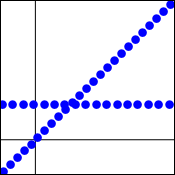## Continuity and Discontinuity

Functions which have the characteristic that their graphs can be drawn without lifting the pencil from the paper are somewhat special, in that they have no funny behaviors. The property which describes this characteristic is called continuity.

### Definition of Continuity at a Point

A function $f(x)$ is continuous at a point where   $x=c$   when the following three conditions are satisfied.

• The function exists at   $x=c$.   (In other words, $f(c)$ is a real number.)
• The limit of the function exists at   $x=c$.   (That is,   $\lim\limits_{x\to c}f(x)$   is a real number.)
• The two values are equal. (That is,   $\lim\limits_{x\to c}f(x)=f(c)$.)

If a function has a hole, the three conditions effectively insist that the hole be filled in with a point to be a continuous function.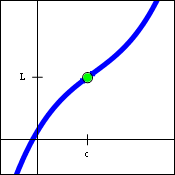### More Definitions

Continuity can also be defined on one side of a point, using a one-sided limit.

• A function $f(x)$ is continuous from the left at the value   $x=c$   when $f(c)$ exists,   $\lim\limits_{x\to c-}f(x)$   exists, and   $\lim\limits_{x\to c-}f(x)=f(c)$.
• A function $f(x)$ is continuous from the right at the value   $x=c$   when $f(c)$ exists,   $\lim\limits_{x\to c+}f(x)$   exists, and   $\lim\limits_{x\to c+}f(x)=f(c)$.

We can also define continuity on an interval.

• A function $f(x)$ is continuous on the open interval   $(a,b)$   if it is continuous at every point   $x=c$   contained in that interval.
• A function $f(x)$ is continuous on the closed interval   $[a,b]$   if it is continuous on the open interval   $(a,b)$,   it is continuous from the right at   $x=a$,   and continuous from the left at   $x=b$.
• A function $f(x)$ is continuous everywhere if it is continuous at every point on the interval   $(-\infty,\infty)$.

Rather than define whether a function is continuous or not, it is more useful to determine where a function is continuous.

### Testing for Continuity

Show that the function   $f(x)=\left\{\matrix{ \dfrac{2x-6}{x-3} & \text{when } x\ne 3 \\ 2 & \text{when } x=3}\right\}$   is continuous at   $x=3$.

 Note that   $f(3)=2$,   by the definition of $f(x)$. Therefore the function exists, and the first condition is met. Also,   $\lim\limits_{x\to 3}\dfrac{2x-6}{x-3}=\lim\limits_{x\to 3}\dfrac{2(x-3)}{x-3}=\lim\limits_{x\to 3}2=2$. The limit exists, and the second condition is met. And this implies   $\lim\limits_{x\to 3}\dfrac{2x-6}{x-3}=f(3)$.   Therefore, $f(x)$ is continuous at   $x=3$. The equality demonstrates the third and last condition.

To prove a function is not continuous, it is sufficient to show that one of the three conditions stated above is not met.

### Types of Discontinuity

When a function is not continuous at a point, then we can say it is discontinuous at that point. There are several types of behaviors that lead to discontinuities.

A removable discontinuity exists when the limit of the function exists, but one or both of the other two conditions is not met. The graphical feature that results is often colloquially called a hole. The first graph below shows a function whose value at   $x=c$   is not defined. The second graph below shows a function which has both a limit and a value at   $x=c$,   but the two values are not equal. This type of function is frequently encountered when trying to find slopes of tangent lines.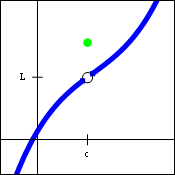An infinite discontinuity exists when one of the one-sided limits of the function is infinite. In other words,   $\lim\limits_{x\to c+}f(x)=\infty$,   or one of the other three varieties of infinite limits. If the two one-sided limits have the same value, then the two-sided limit will also exist. Graphically, this situation corresponds to a vertical asymptote. Many rational functions exhibit this type of behavior.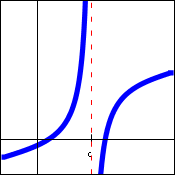A finite discontinuity exists when the two-sided limit does not exist, but the two one-sided limits are both finite, yet not equal to each other. The graph of a function having this feature will show a vertical gap between the two branches of the function. The function   $f(x)=\dfrac{|x|}{x}$   has this feature. The graph below is of a generic function with a finite discontinuity.An oscillating discontinuity exists when the values of the function appear to be approaching two or more values simultaneously. A standard example of this situation is the function   $f(x)=\sin\left(\dfrac{1}{x}\right)$,   pictured below.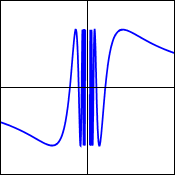It is possible to construct functions with even stranger discontinuities. Often, mathematicians will refer to these examples as "pathological", because their behavior can seem very counterintuitive. One such example is the function   $f(x)=\left\{\matrix{ x & \text{when }x\text{ is rational} \\2 & \text{when }x\text{ is irrational}}\right\}$.   This function can be proven to be continuous at exactly one point only. An approximation of its graph is shown below.